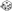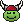Make a Random AD&D Character - Page 4 - Myth-Weavers

 Notices The winners of the first Storycrafting contest have been announced! Congratulations to our grand prize winner, Underleaf, and the runners up!

# Non Sequitur

A place for light-hearted forum games and other threads that don't promote discussion.

# Make a Random AD&D Character

I'm feeling kinda lucky today...

 Race Class Ability Scores Dice Roll: 1d6z d6 Results: 6(6) Dice Roll: 1d9z d9 Results: 8(8) 1 = Human 2 = Elf 3 = Dwarf 4 = Halfling 5 = Gnome 6 = Half-Elf 1 = Fighter 2 = Ranger 3 = Paladin 4 = Cleric 5 = Druid 6 = Thief 7 = Bard 8 = Mage 9 = Illusionist Dice Roll: 3d6z d6 Results: 5, 3, 2 (Total = 10)Strength (10) Dice Roll: 3d6z d6 Results: 6, 5, 4 (Total = 15)Dexterity (15) Dice Roll: 3d6z d6 Results: 5, 3, 6 (Total = 14)Constitution (14) Dice Roll: 3d6z d6 Results: 1, 3, 2 (Total = 6)Intelligence (6) Dice Roll: 3d6z d6 Results: 1, 6, 1 (Total = 8)Wisdom (8) Dice Roll: 3d6z d6 Results: 3, 3, 4 (Total = 10)Charisma (10)

Then again, maybe not. A Half-Elf Mage...with a 6 INT.

I have to warn you, I'm feeling lucky today!

 Race Class Ability Scores Dice Roll: 1d1+6z d1 Results: 1 (Total = 7)(7) Dice Roll: 1d1+9z d1 Results: 1 (Total = 10)(10) 1 = Human 2 = Elf 3 = Dwarf 4 = Halfling 5 = Gnome 6 = Half-Elf 7 = Demi-God 1 = Fighter 2 = Ranger 3 = Paladin 4 = Cleric 5 = Druid 6 = Thief 7 = Bard 8 = Mage 9 = Illusionist 10 = Ranger/Paladin/Bard/Cleric/Druid/Mage/Illusionist Dice Roll: 18d1z d1 Results: 1, 1, 1, 1, 1, 1, 1, 1, 1, 1, 1, 1, 1, 1, 1, 1, 1, 1 (Total = 18)Strength (18) Dice Roll: 18d1z d1 Results: 1, 1, 1, 1, 1, 1, 1, 1, 1, 1, 1, 1, 1, 1, 1, 1, 1, 1 (Total = 18)Dexterity (18) Dice Roll: 18d1z d1 Results: 1, 1, 1, 1, 1, 1, 1, 1, 1, 1, 1, 1, 1, 1, 1, 1, 1, 1 (Total = 18)Constitution (18) Dice Roll: 18d1z d1 Results: 1, 1, 1, 1, 1, 1, 1, 1, 1, 1, 1, 1, 1, 1, 1, 1, 1, 1 (Total = 18)Intelligence (18) Dice Roll: 18d1z d1 Results: 1, 1, 1, 1, 1, 1, 1, 1, 1, 1, 1, 1, 1, 1, 1, 1, 1, 1 (Total = 18)Wisdom (18) Dice Roll: 18d1z d1 Results: 1, 1, 1, 1, 1, 1, 1, 1, 1, 1, 1, 1, 1, 1, 1, 1, 1, 1 (Total = 18)Charisma (18)

Nailed it! Imagine the odds!

Character: Cheaty MacFudgeliar - Strong, brilliant, handsome, nimble, wise, and hale, Cheaty breaks all the rules.Ok, ok...I'll give it another try...

 Race Class Ability Scores Dice Roll: 1d6z d6 Results: 5(5) Dice Roll: 1d9z d9 Results: 8(8) 1 = Human 2 = Elf 3 = Dwarf 4 = Halfling 5 = Gnome 6 = Half-Elf 1 = Fighter 2 = Ranger 3 = Paladin 4 = Cleric 5 = Druid 6 = Thief 7 = Bard 8 = Mage 9 = Illusionist Dice Roll: 3d6z d6 Results: 5, 3, 2 (Total = 10)Strength (10) Dice Roll: 3d6z d6 Results: 4, 5, 1 (Total = 10)Dexterity (10) Dice Roll: 3d6z d6 Results: 4, 6, 3 (Total = 13)Constitution (13) Dice Roll: 3d6z d6 Results: 3, 4, 4 (Total = 11)Intelligence (11) Dice Roll: 3d6z d6 Results: 4, 4, 2 (Total = 10)Wisdom (10) Dice Roll: 3d6z d6 Results: 3, 2, 5 (Total = 10)Charisma (10)

Character: Gnome Mage, Mediokyr MacDeadaverage - This mage dresses in gray has no particular interests, and was a solid C student at wizard school.

 Race Class Ability Scores Dice Roll: 1d6z d6 Results: 2(2) Dice Roll: 1d9z d9 Results: 5(5) 1 = Human 2 = Elf 3 = Dwarf 4 = Halfling 5 = Gnome 6 = Half-Elf 1 = Fighter 2 = Ranger 3 = Paladin 4 = Cleric 5 = Druid 6 = Thief 7 = Bard 8 = Mage 9 = Illusionist Dice Roll: 3d6z d6 Results: 6, 1, 3 (Total = 10)Strength (10) Dice Roll: 3d6z d6 Results: 1, 6, 1 (Total = 8)Dexterity (8) Dice Roll: 3d6z d6 Results: 3, 1, 1 (Total = 5)Constitution (5) Dice Roll: 3d6z d6 Results: 1, 3, 5 (Total = 9)Intelligence (9) Dice Roll: 3d6z d6 Results: 4, 1, 5 (Total = 10)Wisdom (10) Dice Roll: 3d6z d6 Results: 6, 3, 4 (Total = 13)Charisma (13)

An Elf druid who is apparently made of porcelain.

 Race Class Ability Scores Dice Roll: 1d6z d6 Results: 3(3) Dice Roll: 1d9z d9 Results: 9(9) 1 = Human 2 = Elf 3 = Dwarf 4 = Halfling 5 = Gnome 6 = Half-Elf 1 = Fighter 2 = Ranger 3 = Paladin 4 = Cleric 5 = Druid 6 = Thief 7 = Bard 8 = Mage 9 = Illusionist Dice Roll: 3d6z d6 Results: 5, 6, 3 (Total = 14)Strength (14) Dice Roll: 3d6z d6 Results: 5, 3, 4 (Total = 12)Dexterity (12) Dice Roll: 3d6z d6 Results: 3, 2, 4 (Total = 9)Constitution (9) Dice Roll: 3d6z d6 Results: 5, 4, 3 (Total = 12)Intelligence (12) Dice Roll: 3d6z d6 Results: 5, 1, 3 (Total = 9)Wisdom (9) Dice Roll: 3d6z d6 Results: 4, 5, 4 (Total = 13)Charisma (13)

Hmm... a strong dwarf illusionist...

Stupid truth, resisting simplicity.

Well. In spite of my first set actually being *playable*, let's see what I get to play next... after the inevitable TPK because none of my other party members could survive more than 2 combat rounds.

 Race Class Ability Scores Dice Roll: 1d6z d6 Results: 1(1) Dice Roll: 1d9z d9 Results: 4(4) 1 = Human 2 = Elf 3 = Dwarf 4 = Halfling 5 = Gnome 6 = Half-Elf 1 = Fighter 2 = Ranger 3 = Paladin 4 = Cleric 5 = Druid 6 = Thief 7 = Bard 8 = Mage 9 = Illusionist Dice Roll: 3d6z d6 Results: 3, 1, 2 (Total = 6)Strength (6) Dice Roll: 3d6z d6 Results: 6, 5, 6 (Total = 17)Dexterity (17) Dice Roll: 3d6z d6 Results: 6, 5, 1 (Total = 12)Constitution (12) Dice Roll: 3d6z d6 Results: 4, 4, 4 (Total = 12)Intelligence (12) Dice Roll: 3d6z d6 Results: 2, 2, 5 (Total = 9)Wisdom (9) Dice Roll: 3d6z d6 Results: 1, 5, 6 (Total = 12)Charisma (12)

 Race Class Ability Scores Dice Roll: 1d6z d6 Results: 3(3) Dice Roll: 1d9z d9 Results: 8(8) 1 = Human 2 = Elf 3 = Dwarf 4 = Halfling 5 = Gnome 6 = Half-Elf 1 = Fighter 2 = Ranger 3 = Paladin 4 = Cleric 5 = Druid 6 = Thief 7 = Bard 8 = Mage 9 = Illusionist Dice Roll: 3d6z d6 Results: 2, 5, 4 (Total = 11)Strength (11) Dice Roll: 3d6z d6 Results: 3, 6, 3 (Total = 12)Dexterity (12) Dice Roll: 3d6z d6 Results: 1, 6, 6 (Total = 13)Constitution (13) Dice Roll: 3d6z d6 Results: 4, 4, 2 (Total = 10)Intelligence (10) Dice Roll: 3d6z d6 Results: 4, 2, 4 (Total = 10)Wisdom (10) Dice Roll: 3d6z d6 Results: 6, 3, 4 (Total = 13)Charisma (13)

Let's see what happens this time.

Here we go again!
 Race Class Ability Scores Dice Roll: 1d6z d6 Results: 6(6) Dice Roll: 1d9z d9 Results: 5(5) 1 = Human 2 = Elf 3 = Dwarf 4 = Halfling 5 = Gnome 6 = Half-Elf 1 = Fighter 2 = Ranger 3 = Paladin 4 = Cleric 5 = Druid 6 = Thief 7 = Bard 8 = Mage 9 = Illusionist Dice Roll: 3d6z d6 Results: 5, 3, 3 (Total = 11)Strength (11) Dice Roll: 3d6z d6 Results: 6, 6, 1 (Total = 13)Dexterity (13) Dice Roll: 3d6z d6 Results: 1, 3, 1 (Total = 5)Constitution (5) Dice Roll: 3d6z d6 Results: 1, 5, 5 (Total = 11)Intelligence (11) Dice Roll: 3d6z d6 Results: 1, 1, 4 (Total = 6)Wisdom (6) Dice Roll: 3d6z d6 Results: 6, 3, 6 (Total = 15)Charisma (15)

Failure, Half-elf druid with 6 Wis.

Take 2!

 Race Class Ability Scores Dice Roll: 1d6z d6 Results: 1(1) Dice Roll: 1d9z d9 Results: 5(5) 1 = Human 2 = Elf 3 = Dwarf 4 = Halfling 5 = Gnome 6 = Half-Elf 1 = Fighter 2 = Ranger 3 = Paladin 4 = Cleric 5 = Druid 6 = Thief 7 = Bard 8 = Mage 9 = Illusionist Dice Roll: 3d6z d6 Results: 5, 6, 4 (Total = 15)Strength (15) Dice Roll: 3d6z d6 Results: 4, 1, 3 (Total = 8)Dexterity (8) Dice Roll: 3d6z d6 Results: 6, 5, 2 (Total = 13)Constitution (13) Dice Roll: 3d6z d6 Results: 3, 3, 6 (Total = 12)Intelligence (12) Dice Roll: 3d6z d6 Results: 2, 5, 6 (Total = 13)Wisdom (13) Dice Roll: 3d6z d6 Results: 1, 6, 5 (Total = 12)Charisma (12)

 Race Class Ability Scores Dice Roll: 1d6z d6 Results: 3(3) Dice Roll: 1d9z d9 Results: 9(9) 1 = Human 2 = Elf 3 = Dwarf 4 = Halfling 5 = Gnome 6 = Half-Elf 1 = Fighter 2 = Ranger 3 = Paladin 4 = Cleric 5 = Druid 6 = Thief 7 = Bard 8 = Mage 9 = Illusionist Dice Roll: 3d6z d6 Results: 4, 4, 3 (Total = 11)Strength (11) Dice Roll: 3d6z d6 Results: 6, 3, 6 (Total = 15)Dexterity (15) Dice Roll: 3d6z d6 Results: 6, 6, 5 (Total = 17)Constitution (17) Dice Roll: 3d6z d6 Results: 2, 5, 4 (Total = 11)Intelligence (11) Dice Roll: 3d6z d6 Results: 6, 3, 6 (Total = 15)Wisdom (15) Dice Roll: 3d6z d6 Results: 4, 2, 1 (Total = 7)Charisma (7)

A dwarf illusionist...very tough and dexterous, wise but far from being charming.

 Race Class Ability Scores Dice Roll: 1d6z d6 Results: 4(4) Dice Roll: 1d9z d9 Results: 6(6) 1 = Human 2 = Elf 3 = Dwarf 4 = Halfling 5 = Gnome 6 = Half-Elf 1 = Fighter 2 = Ranger 3 = Paladin 4 = Cleric 5 = Druid 6 = Thief 7 = Bard 8 = Mage 9 = Illusionist Dice Roll: 3d6z d6 Results: 3, 2, 2 (Total = 7)Strength (7) Dice Roll: 3d6z d6 Results: 1, 3, 1 (Total = 5)Dexterity (5) Dice Roll: 3d6z d6 Results: 3, 4, 4 (Total = 11)Constitution (11) Dice Roll: 3d6z d6 Results: 6, 6, 6 (Total = 18)Intelligence (18) Dice Roll: 3d6z d6 Results: 5, 5, 3 (Total = 13)Wisdom (13) Dice Roll: 3d6z d6 Results: 1, 1, 4 (Total = 6)Charisma (6)

Molin the halfling thief, barely able to lift a sword or bow, but he sure does get through locks quick.